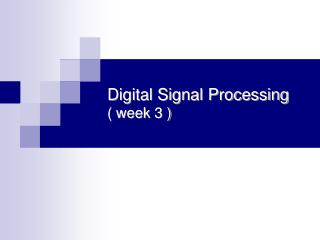DownloadDownload PresentationDigital Signal Processing ( week 3 )

# Digital Signal Processing ( week 3 )

Download Presentation## Digital Signal Processing ( week 3 )

- - - - - - - - - - - - - - - - - - - - - - - - - - - E N D - - - - - - - - - - - - - - - - - - - - - - - - - - -
##### Presentation Transcript

1. Digital Signal Processing( week 3 )

2. Review of Complex Numbers • Representation of Complex Numbers • Catersian plane • real part : x=Re(z) • image part : y=Im(z) • j = Im(z) x Re(z) y (x,y)

3. Review of Complex Numbers • Representation of Complex Numbers • Complex plane • magnitude : r , (denote, |z|) • argument : θ , (denote, arg z) Im(z) θ x Re(z) r y

4. Review of Complex Numbers • Representation of Complex Numbers • Catersian plane ⇔ Complex plane

5. Complex Exponential Signals • representation • a complex-valued function of t • A : the magnitude, |z(t)| = A • (w0t+Φ) : the angle of z(t) • Φ : phase shift • w0 : frequency (rad/sec)

6. Properties • the Rotating Phasor Interpretation • the magnitude : multiply • the angle : add 일 때,

7. Properties • the Rotating Phasor Interpretation 일 때,

8. Properties • Euler Formulas ⇔

9. Properties • Addition of Complex Numbers 일 때,

10. Properties • Addition of Complex Numbers (a) Represent x1(t) and x2(t) by the phasors :

11. Properties • Addition of Complex Numbers (b) Convert both phasors to rectangular form: (c) Add the real parts and the imaginary parts: (d) Convert back to polar form: (e) The final formula

12. Properties • Addition of Complex Numbers

13. Matlab 교재 CD 없는 학생 • ftp://image.dgu.ac.kr • id : dsp • pass : dsp • “DSP \ spfirst.zip”다운로드 • 압축 풀고, • File → Set Path • Add path “/spfirst”

14. Matlab 교재 CD 있는 학생 • Insert CD (in your textbook) & Run Matlab • File → Set Path • Add path “CD/Matlab/spfirst”

15. Matlab • 함수 • UCPLOT( ) • Plot a circle with specified center and radius • ZVECT( ) • Plot vectors in complex z-plane from zFrom to zTo • ZPRINT( ) • Printout complex # in rect and polar form • ZCAT( ) • Plot vectors in z-plane end-to-end

16. Matlab • 예 제 • 각 함수를 이용하여 연습하시오 • zvect( ) • zprint( ) • zcat( ) 1+j j 3-4j ejπ ej2π/3

17. Matlab

18. Matlab

19. Matlab

20. 과 제

21. 과 제

22. 과 제 • 작성한 M 파일과 HWP(DOC) 파일 압축 • 파일명 : 04_학번_성명.zip • 수업시간 끝나기 전까지 • E-mail : young220 @ paran.com

23. 수고 하셨습니다